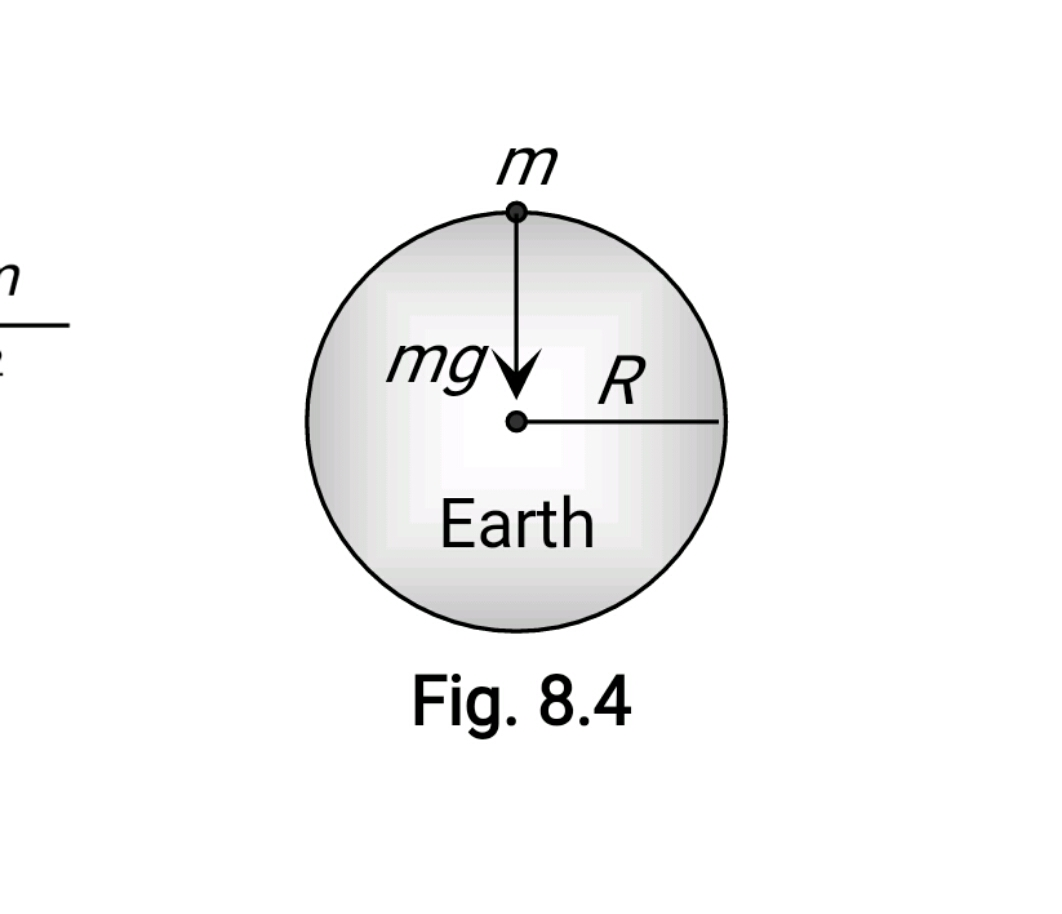#### A spherical planet far out in space has a mass M0 and diameter D0. A particle of mass m falling freely near the surface of this planet will experience an acceleration due to gravity which is equal to Option 1) GM0/D02   Option 2) 4mGM0/D02   Option 3) 4GM0/D02   Option 4) GmM0/D02As we learnt in

Acceleration due to gravity (g) -

Force extended by earth on a body is gravity.

Formula:gravitydensity of earthRadius of earth

- wherein

It's average value ison the surface of earthAcceleration due to gravityOption 1)This is incorrect option

Option 2)This is incorrect option

Option 3)This is correct option

Option 4)This is incorrect option